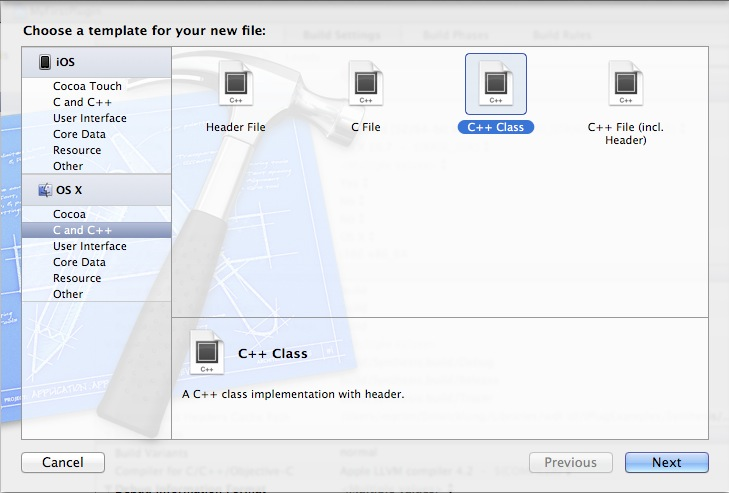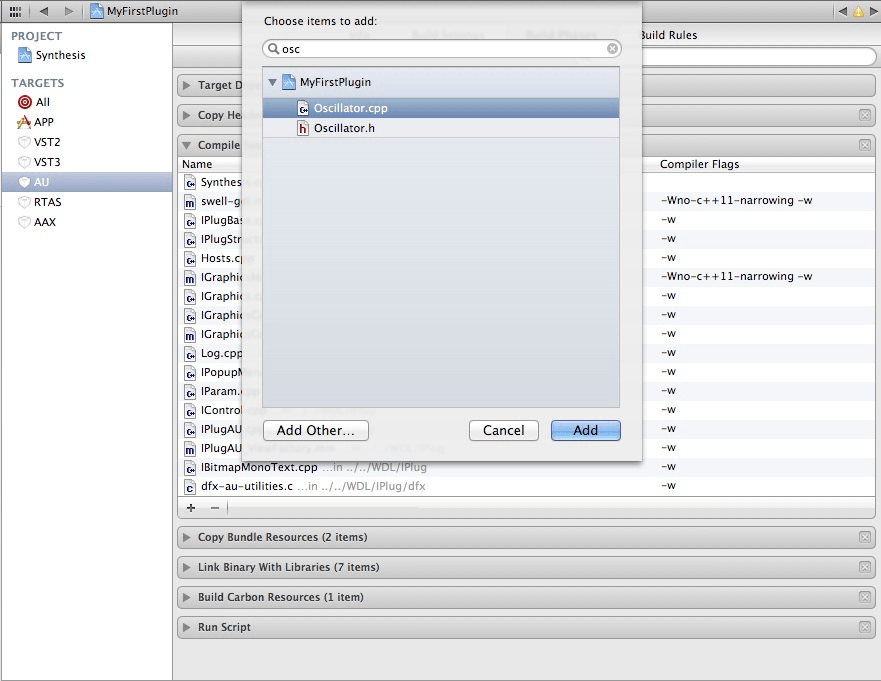# Making Audio Plugins Part 8: Synthesizing Waveforms

After all this GUI stuff it’s time for some audio programming. First we’ll generate the classic Sine, Saw, Square and Triangle waveforms.
Let’s begin by running the duplicate script:

``````./duplicate.py DigitalDistortion/ Synthesis YourName
``````

Again, you’ll have to go to ProductSchemeEdit Scheme… and change “Run” so that it starts REAPER64.app with your reaper-project.RPP (as described earlier). If Reaper complains about the AU not being found, change the names and IDs in resource.h, or remove the DigitalDistortion.component.

## Creating the Oscillator Class

All of this will be about DSP, but we will not just write our code into the `ProcessDoubleReplacing` function. Instead, we will create an `Oscillator` class. It will be called from `ProcessDoubleReplacing` and will fill buffers with `double` values for the current waveform. To generate the waveforms, we’ll first take the most intuitive approach. We will then see the disadvantages and find a better-sounding way.
Create a new Class by going to FileNewFile…:Call it Oscillator.
Now make sure Oscillator.cpp gets compiled when we build. Go to your project settings, select a target (e.g. AU) and click Build Phases. Click the plus button below Compile Sources and add the .cpp file (you’ll have to do this for every target you need):Let’s write the header first. Put this between the `#define` and `#endif` in Oscillator.h:

``````#include <math.h>

enum OscillatorMode {
OSCILLATOR_MODE_SINE,
OSCILLATOR_MODE_SAW,
OSCILLATOR_MODE_SQUARE,
OSCILLATOR_MODE_TRIANGLE
};

class Oscillator {
private:
OscillatorMode mOscillatorMode;
const double mPI;
double mFrequency;
double mPhase;
double mSampleRate;
double mPhaseIncrement;
void updateIncrement();
public:
void setMode(OscillatorMode mode);
void setFrequency(double frequency);
void setSampleRate(double sampleRate);
void generate(double* buffer, int nFrames);
Oscillator() :
mOscillatorMode(OSCILLATOR_MODE_SINE),
mPI(2*acos(0.0)),
mFrequency(440.0),
mPhase(0.0),
mSampleRate(44100.0) { updateIncrement(); };
};
``````

We are using an `enum` to indicate which waveform the oscillator is generating. Here it defaults to a sine wave, but this can be changed using the `setMode` member function. Calculating π this way is more portable than using the `M_PI` constant.
The Oscillator stores the frequency, phase and sample rate. Just to be clear, the phase is the value that will change all the time to indicate where in the waveform cycle the oscillator currently is. The phase increment is the amount that’s added to the phase every sample.
Finally, there’s more setter functions (for frequency and sample rate) and, most importantly, `generate`. This is the function that takes a buffer of doubles and fills it with sample values.

Let’s add the implementation of the setter functions (in Oscillator.cpp):

``````void Oscillator::setMode(OscillatorMode mode) {
mOscillatorMode = mode;
}

void Oscillator::setFrequency(double frequency) {
mFrequency = frequency;
updateIncrement();
}

void Oscillator::setSampleRate(double sampleRate) {
mSampleRate = sampleRate;
updateIncrement();
}

void Oscillator::updateIncrement() {
mPhaseIncrement = mFrequency * 2 * mPI / mSampleRate;
}
``````

The `mPhaseIncrement` depends on both `mFrequency` and `mSampleRate`, so it has to be updated everytime one of the two is changed. We could calculate it every sample, but of course it’s far more efficient to do it here.
Add the implementation for `generate`:

``````void Oscillator::generate(double* buffer, int nFrames) {
const double twoPI = 2 * mPI;
switch (mOscillatorMode) {
case OSCILLATOR_MODE_SINE:
// ...
break;
case OSCILLATOR_MODE_SAW:
// ...
break;
case OSCILLATOR_MODE_SQUARE:
// ...
break;
case OSCILLATOR_MODE_TRIANGLE:
// ...
break;
}
}
``````

This function will be called everytime `ProcessDoubleReplacing` is called. We’re using a `switch` to use the right code for whatever waveform is currently selected.

## Generating Waveforms

The code for generating a sine wave is quite simple:

``````case OSCILLATOR_MODE_SINE:
for (int i = 0; i < nFrames; i++) {
buffer[i] = sin(mPhase);
mPhase += mPhaseIncrement;
while (mPhase >= twoPI) {
mPhase -= twoPI;
}
}
break;
``````

Note that we’re not working with `mFrequency` and `mSampleRate` here. We’re just incrementing `mPhase` and make sure it stays between `0` and `twoPI`. The only more complex operation is the call to the C `sin()` function, which on many systems will be calculated on a hardware level.

Here’s the code for the saw wave:

``````case OSCILLATOR_MODE_SAW:
for (int i = 0; i < nFrames; i++) {
buffer[i] = 1.0 - (2.0 * mPhase / twoPI);
mPhase += mPhaseIncrement;
while (mPhase >= twoPI) {
mPhase -= twoPI;
}
}
break;
``````

The interesting part is – again – the line of code that’s writing into the buffer. When I see formulas like these, I like to decompose them:

• `mPhase` goes from `0` upwards, and jumps back to `0` when it reaches `twoPI`.
• So `(mPhase / twoPI)` goes from `0` upwards and jumps back to `0` when it reaches `1`.
• This means that `(2.0 * mPhase / twoPI)` goes from `0` up and jumps back at `2`.
• When `mPhase` is `0`, the expression `1.0 - (2.0 * mPhase / twoPI)` is `1`. While `mPhase` goes upwards, the expression goes downwards and jumps back to `1` when it reaches `-1`.

So we have a downwards saw wave!
The lower part that’s dealing with `mPhase` is duplication that could be avoided, but in that case we would have to take the `switch` statement into the loop. This would also prevent duplicating the `for` statement, but the code would `switch` more often than neccessary.
In most programming scenarios, we would prefer brevity and readability over performance. DSP code that’s executed 44100 or 96000 times per second can be an exception to this rule. But be aware that the compiler will optimize a lot behind the scenes and what feels like “a lot of work” to you (the programmer), may be very trivial compared to other areas you’re not thinking about.

Next is the square wave:

``````case OSCILLATOR_MODE_SQUARE:
for (int i = 0; i < nFrames; i++) {
if (mPhase <= mPI) {
buffer[i] = 1.0;
} else {
buffer[i] = -1.0;
}
mPhase += mPhaseIncrement;
while (mPhase >= twoPI) {
mPhase -= twoPI;
}
}
break;
``````

You’re already familiar with the lower part. Every cycle is `twoPI` long, so the `if` statement causes the first half of every cycle to have values of `1`, and the second half to have values of `-1`. So there’s a very sudden jump when `mPhase` becomes greater than `mPI`. That’s a square wave.

The triangle wave is just a little more complex:

``````case OSCILLATOR_MODE_TRIANGLE:
for (int i = 0; i < nFrames; i++) {
double value = -1.0 + (2.0 * mPhase / twoPI);
buffer[i] = 2.0 * (fabs(value) - 0.5);
mPhase += mPhaseIncrement;
while (mPhase >= twoPI) {
mPhase -= twoPI;
}
}
break;
``````

If you decompose `-1.0 + (2.0 * mPhase / twoPI)` like I did above, you’ll notice that it’s the inverse of the above saw wave: It’s an upwards saw wave.
Let’s go from here: Taking absolute values (`fabs`) of the upwards saw wave means that all values below `0` will be inverted (flipped around the x axis). This means that the values will go up and down. Subtracting `0.5` centers the waveform around `0`. Multiplying by `2.0` makes the values go between `-1` to `+1`. We have a triangle wave.

Let’s use our oscillator! Include Oscillator.h and add an `Oscillator` member to your `Synthesis` class:

``````// ...
#include "Oscillator.h"

class Synthesis : public IPlug
{
// ...
private:
double mFrequency;
void CreatePresets();
Oscillator mOscillator;
};
``````

We also renamed `mThreshold` to `mFrequency`.
In Synthesis.cpp, rename all instances of `Threshold` with `Frequency`. Now change the parameter initialization inside the constructor:

``````GetParam(kFrequency)->InitDouble("Frequency", 440.0, 50.0, 20000.0, 0.01, "Hz");
GetParam(kFrequency)->SetShape(2.0);
``````

We’re re-using the knob for testing our class. We’ll be able to change the oscillator’s frequency between 50 Hz and 20 kHz (the default will be 440 Hz).
Change the `createPresets` member function:

``````void Synthesis::CreatePresets() {
MakePreset("clean", 440.0);
}
``````

Inside `Reset`, we have to tell the oscillator what sample rate is being used:

``````void Synthesis::Reset()
{
TRACE;
IMutexLock lock(this);
mOscillator.setSampleRate(GetSampleRate());
}
``````

If we didn’t do this and the oscillator had the wrong sample rate, it would still generate the same waveforms, but at the wrong frequencies. `GetSampleRate` is a member function that’s inherited from the `IPlugBase` class.

We have to edit `OnParamChange` as well, so the oscillator’s frequency can be changed using the knob.

``````void Synthesis::OnParamChange(int paramIdx)
{
IMutexLock lock(this);

switch (paramIdx)
{
case kFrequency:
mOscillator.setFrequency(GetParam(kFrequency)->Value());
break;

default:
break;
}
}
``````

Finally, `ProcessDoubleReplacing` has to use the oscillator:

``````void Synthesis::ProcessDoubleReplacing(double** inputs,
double** outputs,
int nFrames) {
// Mutex is already locked for us.

double *leftOutput = outputs;
double *rightOutput = outputs;

mOscillator.generate(leftOutput, nFrames);

// Copy left buffer into right buffer:
for (int s = 0; s < nFrames; ++s) {
rightOutput[s] = leftOutput[s];
}
}
``````

Basically we’re letting `mOscillator` fill the left channel buffer. Then we copy everything into the right channel buffer.
Let’s hear how it sounds! Run the VST2 or AU target. If you get linker errors, make sure you added Oscillator.cpp to the Compile Sources phase.
Once it’s running, you’ll hear a steady tone. Turn the knob and the frequency will change. Now change the initial value of `mOscillatorMode` in Oscillator.h, by modifying the constructor’s initializer list:

``````Oscillator() :
mOscillatorMode(OSCILLATOR_MODE_SAW),
mPI(2*acos(0.0)),
mFrequency(440.0),
mPhase(0.0),
mSampleRate(44100.0) { updateIncrement(); };
``````

Run again and you’ll hear a sharper tone. Try `OSCILLATOR_MODE_SQUARE` and `OSCILLATOR_MODE_TRIANGLE`, too. Note the different timbres and turn the frequency knob. For all waveforms except the sine, you’ll hear that once you get into high frequencies, strange noises appear. There are additional tones, even below the base frequency. They sound inharmonic and when you turn the knob up and down, they move in the opposite direction!

## Aliasing

If you look at the code for the square wave, you’ll notice that every time `mPhase` becomes greater than `mPI`, the waveform will jump from the positive max to the negative max, all from one sample to the next. The opposite jump happens when `twoPI` is subtracted from mPhase and it becomes less than `mPI` again. Generally, sudden jumps in a waveform mean that there’s a lot of high frequency content. Imagine somebody told you to construct this jump using as many sine waves as you like, but only sine waves. Given the generally round shape of sine waves, you can imagine how you’d need a lot of sine waves with a very high frequency. You actually need an infinite number of sine waves with frequencies going towards infinity to create a perfect square, saw or triangle wave.

In computers, everything is finite. You have a limited amount of disk space and RAM, so when you record one second of audio, your computer can only use a finite number of values to save it. This number (called the Sample Rate) can be any value, but is often 44100, 48000 or 96000 samples per second. An audio signal stored using a finite number of samples per second is called discrete.
To describe a signal that’s oscillating between +1 and -1, you need at the very least two samples per cycle: one with the value +1 and one with the value -1. So if you have 44100 samples per second, the maximum frequency you can describe is 22050 Hz (see Nyquist frequency).

So, it’s not possible to describe a perfect square, saw or triangle wave in a discrete time signal. If we try to do it (by generating the sharp jumps in the waveform), we will get aliasing effects. For more information, click here.

How can we generate the best, alias-free wave for a given sample rate? “Best” meaning “closest to the shape we calculated above”.
The Nyquist frequency is a constraint that’s expressed in the frequency domain. It doesn’t say “Your waveform shouldn’t have spikes that are steeper than X”. It says “Your signal shouldn’t have frequencies above X Hz”. So we need to shift our work to the frequency domain. We will do that in a future post, but in the next post we’ll look at how we can receive incoming MIDI data.